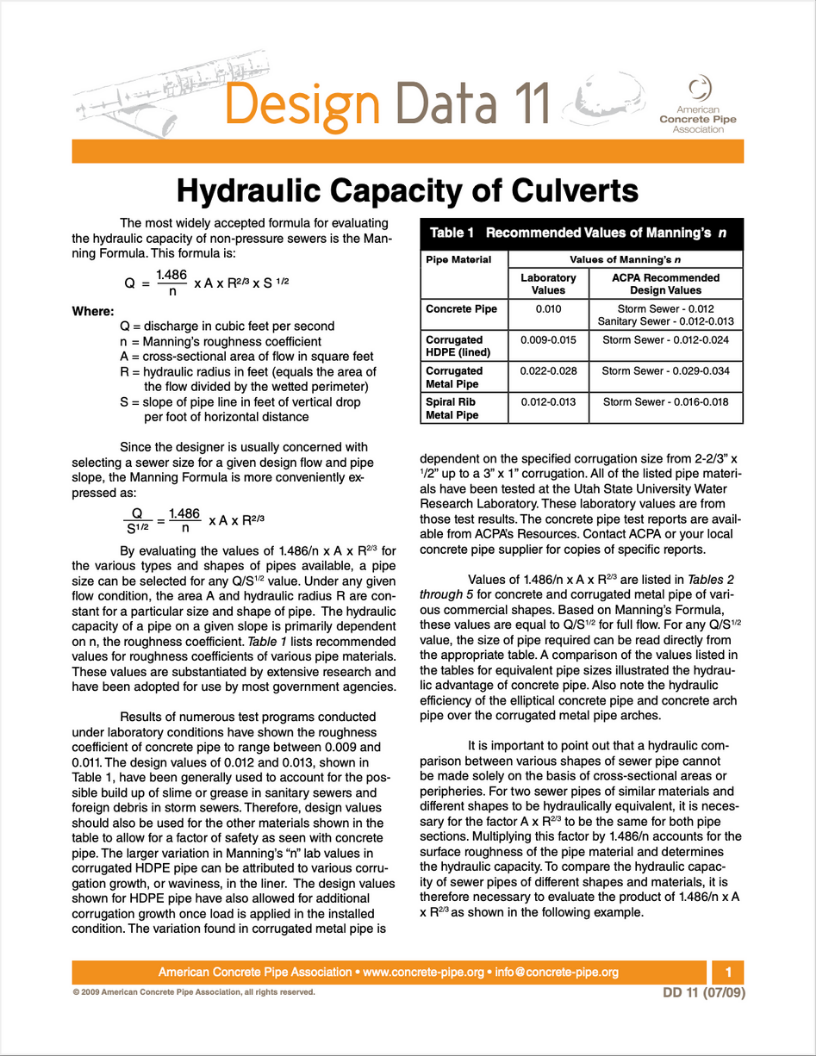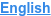Design Data

# Hydraulic Capacity of Culverts

##Design Data 11:  Hydraulic Capacity of Culverts

The most widely accepted formula for evaluating the hydraulic capacity of non-pressure sewers is the Manning Formula.

• Q = discharge in cubic feet per second
• n = Manning’s roughness coefficient
• A = cross-sectional area of flow in square feet
• R = hydraulic radius in feet (equals the area of the flow divided by the wetted perimeter)
• S = slope of pipe line in feet of vertical drop per foot of horizontal distance